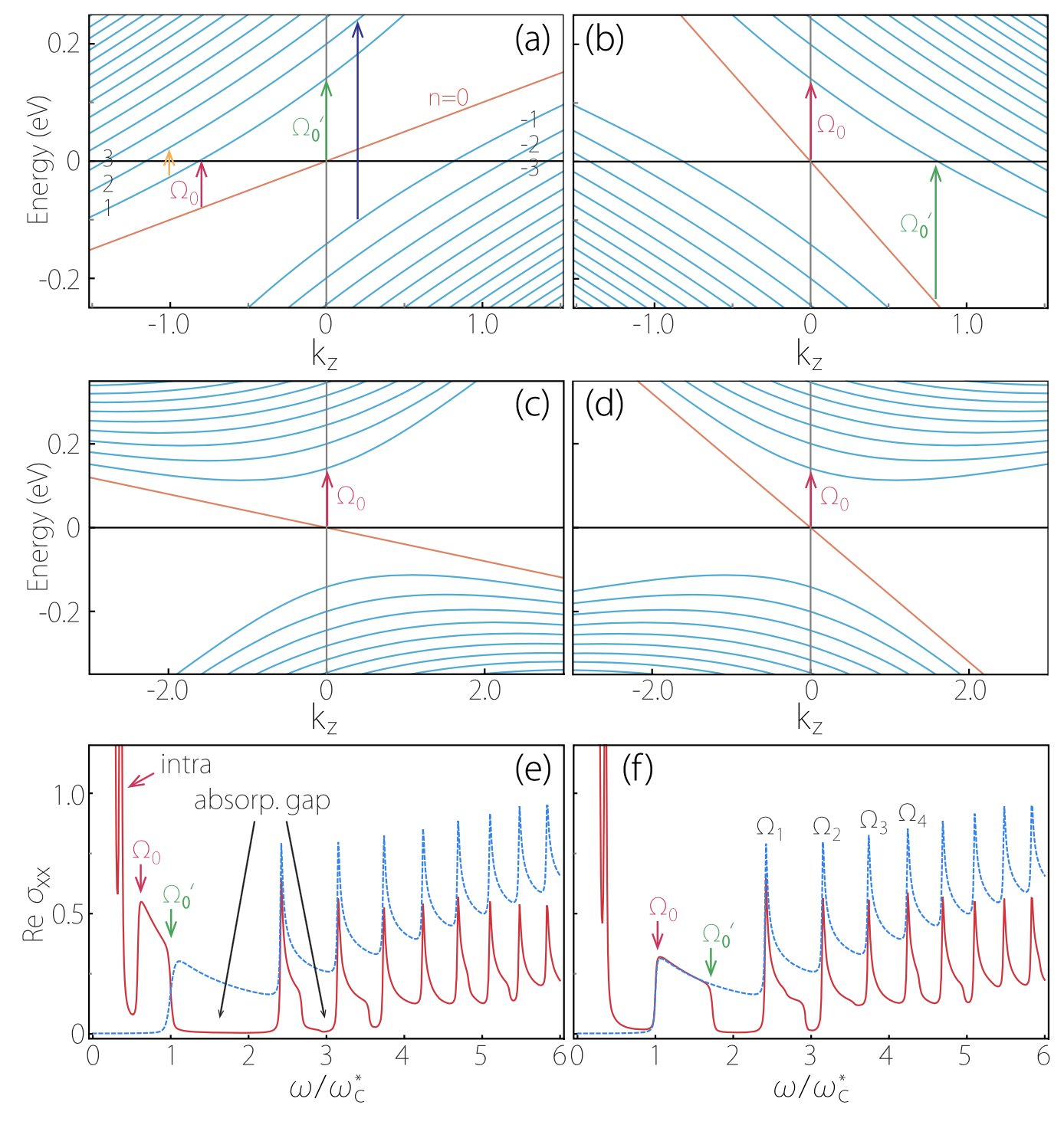# An important progress in Type-II Weyl Semimetal has been made by School of Physics, Beijing Institute of Technology

Sep.19 2016Figure
(a) LL squeezing factor α3/2 plotted versus ^w on a unit sphere. (Upper): Conventional Weyl node with w/v0=0.9. (Lower): Type-II node with w/v0=1.2, in which the two red loops mark the critical angle where the LLs collapse. (b) Semiclassical orbit transforms from closed orbit at β<1 to open trajectory at β>1. Here kz=0, E=0.1eV, and the wave vectors are in units of 0.1eV/v0.Figure
LL dispersion along kz for (a) a type-II Weyl node (w/v0=2) and (c) a conventional Weyl node (w/v0=0.6), both with ^w along the B field (w>0). (b) and (d) are the same as (a) and (c), respectively, but with ^w antiparallel to the B field (w<0). Arrows in (a) mark some representative optical transitions. (e) Reσxx(ω) plotted for the type-II (red solid curve) and conventional Weyl node (blue dashed curve) corresponding to (a) and (c), respectively, in units of e2/(2πB). (f) is the same as (e) but with a reversed field direction, corresponding to (b) and (d). Here β=0, ωc=0.14eV, μ=0eV, and kz is in units of 0.1eV/v0. In (e) and (f), kBT and the scattering rate Γ are set as 0.01ωc.

We show several distinct signatures in the magnetoresponse of type-II Weyl semimetals. The energy tilt tends to squeeze the Landau levels (LLs), and, for a type-II Weyl node, there always exists a critical angle between the B field and the tilt, at which the LL spectrum collapses, regardless of the field strength. Before the collapse, signatures also appear in the magneto-optical spectrum, including the invariable presence of intraband peaks, the absence of absorption tails, and the special anisotropic field dependence.

>> Detail